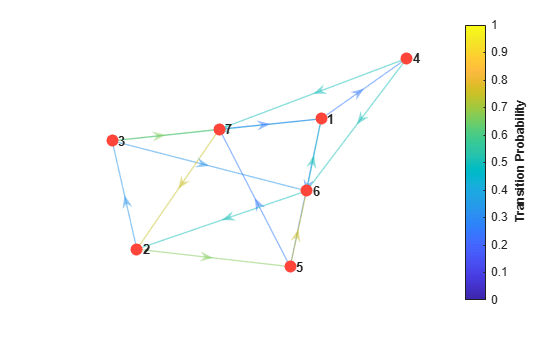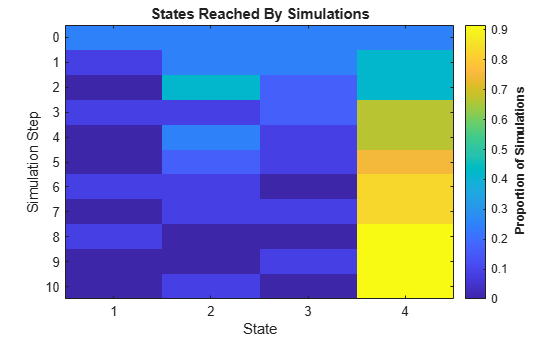# simulate

Simulate Markov chain state walks

## Syntax

``X = simulate(mc,numSteps)``
``X = simulate(mc,numSteps,'X0',x0)``

## Description

example

``X = simulate(mc,numSteps)` returns data `X` on random walks of length `numSteps` through sequences of states in the discrete-time Markov chain `mc`.`

example

``X = simulate(mc,numSteps,'X0',x0)` optionally specifies the initial state of simulations `x0`.`

## Examples

collapse all

Consider this theoretical, right-stochastic transition matrix of a stochastic process.

`$P=\left[\begin{array}{ccccccc}0& 0& 1/2& 1/4& 1/4& 0& 0\\ 0& 0& 1/3& 0& 2/3& 0& 0\\ 0& 0& 0& 0& 0& 1/3& 2/3\\ 0& 0& 0& 0& 0& 1/2& 1/2\\ 0& 0& 0& 0& 0& 3/4& 1/4\\ 1/2& 1/2& 0& 0& 0& 0& 0\\ 1/4& 3/4& 0& 0& 0& 0& 0\end{array}\right].$`

Create the Markov chain that is characterized by the transition matrix P.

```P = [ 0 0 1/2 1/4 1/4 0 0 ; 0 0 1/3 0 2/3 0 0 ; 0 0 0 0 0 1/3 2/3; 0 0 0 0 0 1/2 1/2; 0 0 0 0 0 3/4 1/4; 1/2 1/2 0 0 0 0 0 ; 1/4 3/4 0 0 0 0 0 ]; mc = dtmc(P);```

Plot a directed graph of the Markov chain. Indicate the probability of transition by using edge colors.

```figure; graphplot(mc,'ColorEdges',true);```Simulate a 20-step random walk that starts from a random state.

```rng(1); % For reproducibility numSteps = 20; X = simulate(mc,numSteps)```
```X = 21×1 3 7 1 3 6 1 3 7 2 5 ⋮ ```

`X` is a 21-by-1 matrix. Rows correspond to steps in the random walk. Because `X(1)` is `3`, the random walk begins at state 3.

Visualize the random walk.

```figure; simplot(mc,X);```Create a four-state Markov chain from a randomly generated transition matrix containing eight infeasible transitions.

```rng('default'); % For reproducibility mc = mcmix(4,'Zeros',8);```

`mc` is a `dtmc` object.

Plot a digraph of the Markov chain.

```figure; graphplot(mc);```State `4` is an absorbing state.

Run three 10-step simulations for each state.

```x0 = 3*ones(1,mc.NumStates); numSteps = 10; X = simulate(mc,numSteps,'X0',x0);```

`X` is an 11-by-12 matrix. Rows corresponds to steps in the random walk. Columns 1–3 are the simulations that start at state 1; column 4–6 are the simulations that start at state 2; columns 7–9 are the simulations that start at state 3; and columns 10–12 are the simulations that start at state 4.

For each time, plot the proportions states that are visited over all simulations.

```figure; simplot(mc,X)```## Input Arguments

collapse all

Discrete-time Markov chain with `NumStates` states and transition matrix `P`, specified as a `dtmc` object. `P` must be fully specified (no `NaN` entries).

Number of discrete time steps in each simulation, specified as a positive integer.

Data Types: `double`

### Name-Value Pair Arguments

Specify optional comma-separated pairs of `Name,Value` arguments. `Name` is the argument name and `Value` is the corresponding value. `Name` must appear inside quotes. You can specify several name and value pair arguments in any order as `Name1,Value1,...,NameN,ValueN`.

Example: `'X0',[1 0 2]` specifies simulating three times, the first simulation starts in state 1 and the final two start in state 3.

Initial states of simulations, specified as the comma-separated pair consisting of `'X0'` and a vector of nonnegative integers of `NumStates` length. `X0` provides counts for the number of simulations to begin in each state. The total number of simulations (`numSims`) is `sum(X0)`.

The default is a single simulation beginning from a random initial state.

Example: `'X0',[10 10 0 5]`

Data Types: `double`

## Output Arguments

collapse all

Indices of states visited during the simulations, returned as a `(1 + numSteps)`-by-`numSims` numeric matrix of positive integers. The first row contains the initial states. Columns, in order, are all simulations beginning in the first state, then all simulations beginning in the second state, and so on.

## Tips

• To start `n` simulations from state `k`, use:

```X0 = zeros(1,NumStates); X0(k) = n;```

• To visualize the data created by `simulate`, use `simplot`.

### Functions

Introduced in R2017b